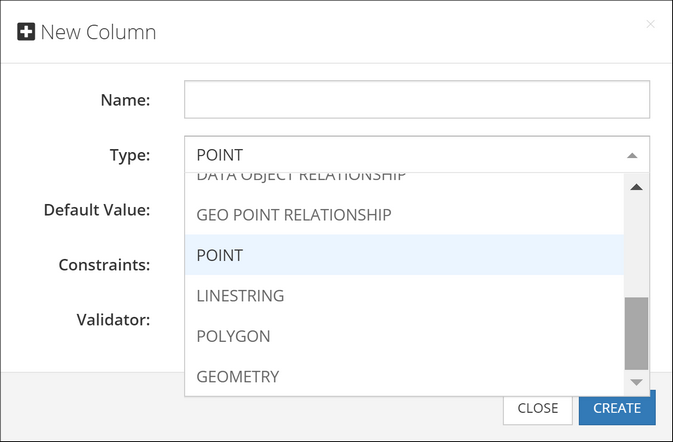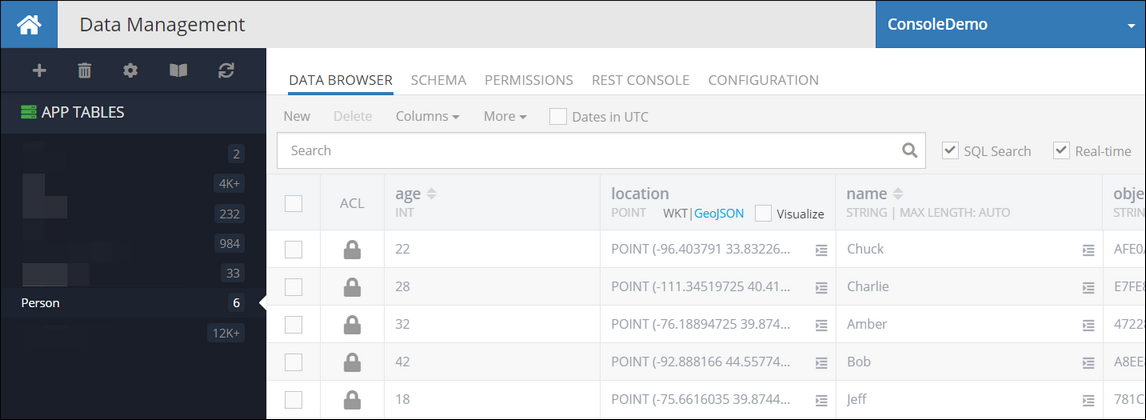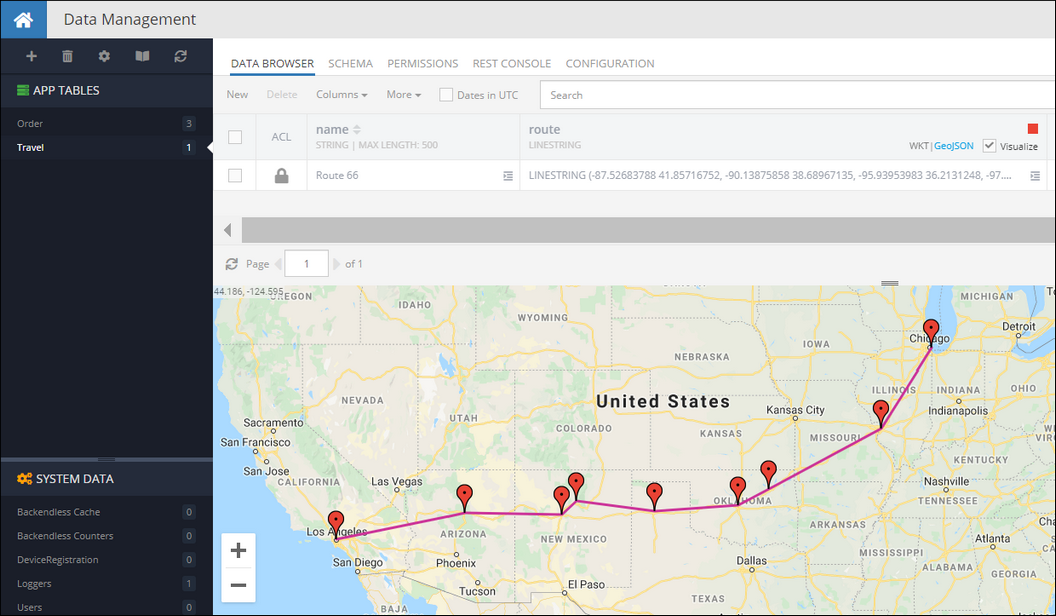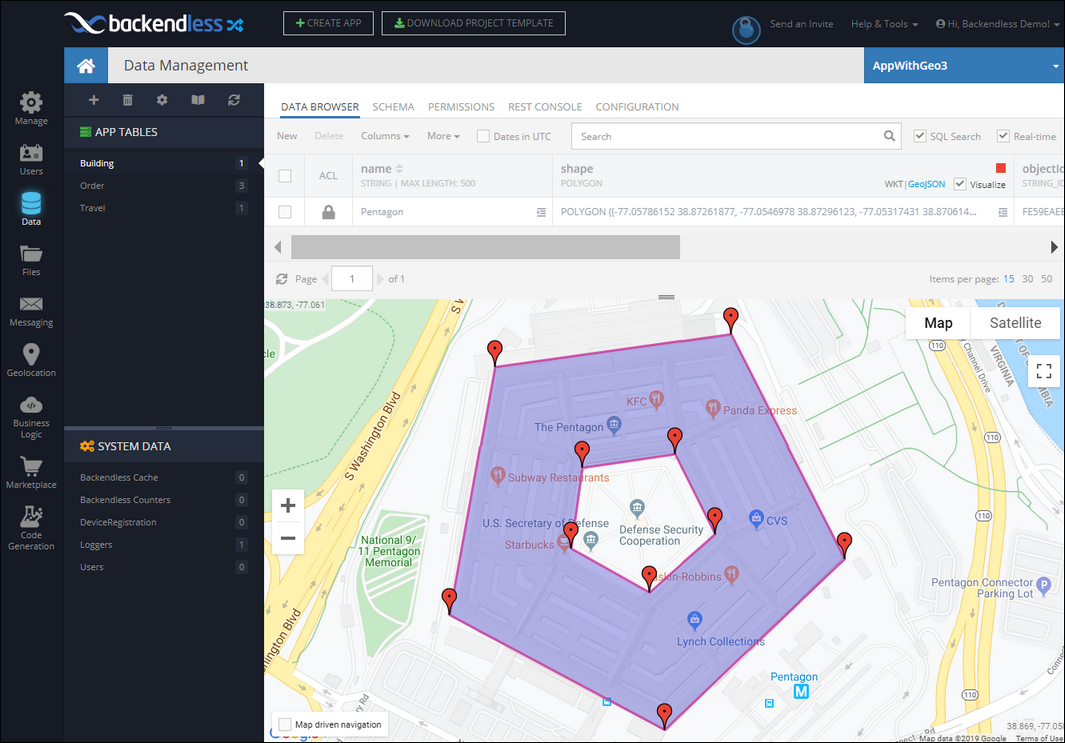# Spatial Data Types¶

Backendless database supports the following spatial data types:

• `POINT` - represents a single point/location in coordinate space. For the points representing locations on a map, the coordinates are longitude and latitude.
• `LINESTRING` - represents a geometry consisting of a collection of points with linear interpolation between them. For example, a linestring can represent a delivery route.
• `POLYGON` - a closed geometrical shape consisting of a single exterior boundary and zero or more interior boundaries, also referred to as holes.

Additionally, Backendless supports a "parent" data type called `GEOMETRY`. This is the base type which can accommodate any of the data types listed above.Spatial data in Backendless can be represented either in the WKT (Well-Known Text) or the GeoJSON formats. Backendless console supports both of these formats for entering new data or updating existing spatial values. Additionally, Backendless SDKs provide built-in classes which make it easier to work with the spatial data for all database operations, including creating, retrieving, updating and deleting spatial data.

## POINT¶

The `POINT` type is used to represent a single point identified by two coordinates in a coordinates space. The coordinates are named X and Y, however, Backendless also handles these coordinates as longitude (X) and latitude (Y) to represent locations on a map. The WKT representation of this type is:

``````POINT (longitude latitude)
``````

or

``````POINT (x y)
``````

for example, the `POINT` below points to Dallas, TX

``````POINT (-96.7553535 32.8656106)
``````

The GeoJSON format for the `POINT` values is:

``````{
"type": "Point",
"coordinates": [
longitude or X,
latitude or Y
]
}
``````

A `POINT` value stored in the database is represented by the `com.backendless.persistence.Point` class in the client application. The class provides access to the point coordinates (`x` and `y`) which can also represent longitude and latitude in cases when the point identifies a location on a map. The class has the constructor and methods listed below. Notice that all `set` methods return the current `Point` object, which allows for convenient "chaining" for property assignments: `pointInstance.setX( value ).setY( value )`:

``````// creates a new instance of Point
public Point()

// creates a Point object from a WKT definition
public static Point fromWKT( String wktPoint )

// creates a Point object from a GeoJSON definition
public static Point fromGeoJSON( String geoJSON )

// retrieves the X coordinate of the point (same as longitude)
public double getX()

// retrieves the Y coordinate of the point (same as latitude)
public double getY()

// retrieves the longitude coordinate of the point (same as x)
public double getLongitude()

// retrieves the latitude coordinate of the point (same as y)
public double getLatitude()

// sets the x coordinate of the point (same as longitude)
public Point setX( double x )

// sets the y coordinate of the point (same as latitude)
public Point setY( double y )

// sets the longitude coordinate of the point (same as x)
public Point setLongitude( double x )

// sets the latitude ccordinatte of the point (same as y)
public Point setLatitude( double y )

// converts this Point object into its WKT representation
public String asWKT()

// converts this Point object into its GeoJSON representation
public String asGeoJSON()

// checks this Point object with another object for equality
public boolean equals( Object o )

// calculates a hash code of this Point object based on the coordinate values and the SRS code.
public int hashCode()
``````

Consider the following example. The objects shown below contain a geometry column/property called `location`. The type of the column is `POINT`:The following code retrieves the first object from the table. Notice how the geometry property is accessed. Backendless automatically converts `POINT` data type to an instance of the `Point` class:

``````Backendless.Data.of( Person.class ).findFirst( new AsyncCallback<Person>()
{
public void handleResponse( Person person )
{

Point location = person.getLocation();

double locationLatitude = location.getLatitude();
double locationLongitude = location.getLongitude();
}

public void handleFault( BackendlessFault error )
{
}
} );
``````
``````Backendless.Data.of<Person>(Person::class.java).findFirst(object : AsyncCallback<Person>
{
override fun handleResponse(person: Person) {

val location = person.location

val locationLatitude = location.latitude
val locationLongitude = location.longitude
}

override fun handleFault(error: BackendlessFault) {}
})
``````

## LINESTRING¶

The `LINESTRING` type is used to represent geometries composed of multiple `POINT` values with linear interpolation between each two consecutive points. The WKT representation of this type is:

``````LINESTRING (lon1 lat1, lon2 lat2, lon3 lat3, lon4 lat4)
``````

or

``````LINESTRING (x1 y1, x2 y2, x3 y3, x4 y4)
``````

for example, the `LINESTRING` below identifies the main stops of the historic Route 66:

``````LINESTRING (-87.52683788 41.85716752, -90.13875858 38.68967135, -95.93953983 36.2131248, -97.49959842 35.53656483, -101.8282117 35.26791494, -105.87118045 35.72083154, -106.61825076 35.14794417, -111.63900272 35.20182535, -118.24178592 34.07195769)
``````

The GeoJSON format for the `LINESTRING` values is:

``````{
"type": "LineString",
"coordinates": [
[
lon1 or x1,
lat1 or y1
],
[
lon2 or x2,
lat2 or x2
],
[
lon3 or x3,
lat3 or y3
],
[
lon4 or x4,
lat4 or y4
]
]
}
``````

Database values of this type are represented by the `com.backendless.persistence.LineString` class in the client application. The class provides access to the `Point` objects making up the linestring. The class has the constructors and methods as listed below. Notice that all `set` method return the current `LineString` object, which allows for convenient "chaining": `lineStringInstance.setPoints( value ).asWKT()`:

``````// creates a new instance of LineString
public LineString(List<Point> points)

// creates a LineString object from a WKT definition
public static LineString fromWKT( String wktLineString )

// creates a LineString object from a GeoJSON definition
public static LineString fromGeoJSON( String geoJSON )

// returns a collection of Point objects making up this LineString
public List<Point> getPoints()

// sets a collection of Point objects to define the LineString
public LineString setPoints(List<Point> points)

// converts this LineString object into its WKT representation
public String asWKT()

// converts this LineString object into its GeoJSON representation
public String asGeoJSON()

// checks this LineString object with another object for equality
public boolean equals( Object o )

// calculates a hash code of this LineString object based on the coordinates of all Points and the SRS code.
public int hashCode()
``````

Consider the following example. The `Travel` table has an object identifying a travel route. The `route` column is of the `LINESTRING` type, its value is visualized in the map in the screenshot below:The following code retrieves the `Travel` object from the table, gets its `route` property (which is a `LineString`) and accesses the points making up the linestring:

``````DataQueryBuilder dataQuery = DataQueryBuilder.create();
dataQuery.setWhereClause( "name = 'Route 66'" );
Backendless.Data.of( "Travel" ).find( dataQuery, new AsyncCallback<List<Map>>()
{
@Override
public void handleResponse( List<Map> response )
{
Map travelRoute = response.get( 0 );
String routeName = (String) travelRoute.get( "name" );
LineString routeDefinition = (LineString) travelRoute.get( "route" );
List<Point> points = routeDefinition.getPoints();
}

@Override
public void handleFault( BackendlessFault fault )
{

}
} );
``````
``````val dataQuery = DataQueryBuilder.create()
dataQuery.whereClause = "name = 'Route 66'"
Backendless.Data.of("Travel").find(dataQuery, object : AsyncCallback<List<Map<Any?, Any?>>> {
override fun handleResponse(response: List<Map<Any?, Any?>>) {
val travelRoute = response
val routeName = travelRoute["name"] as String?
val routeDefinition = travelRoute["route"] as LineString?
val points = routeDefinition?.points
}

override fun handleFault(fault: BackendlessFault) {

}
})
``````

## POLYGON¶

Value of the `POLYGON` type is a figure that is described by a number of `LINESTRING` values connected to form a single continuous exterior boundary. Additionally, a Polygon may  contain zero or more interior boundaries, where each interior boundary defines a hole in the Polygon. The WKT representation of this type is:

``````POLYGON ((lon1 lat1, lon2 lat2, lon3 lat3),
(hole-lon1 hole-lat1, hole-lon2 hole-lat2, hole-lon3 hole-lat3),
(...),(...))
``````

or

``````POLYGON ((x1 y1, x2 y2, x3 y3),
(hole-x1 hole-y1, hole-x2 hole-y2, hole-x3 hole-y3),
(...),(...))
``````

where the first group of coordinates defines the exterior boundary and all subsequent groups defines the holes. The first group is mandatory.

for example, the `POLYGON` below identifies the outline of The Pentagon - the US Department of Defense headquarters. It also includes a hole - which is the inner plaza.

``````POLYGON ((-77.05781934 38.87248788,
-77.05474017 38.87287211,
-77.0533025 38.8706001,
-77.05556629 38.86883758,
-77.05848453 38.87002374,
-77.05781934 38.87248788),
(-77.05669282 38.87156906,
-77.05551265 38.87170271,
-77.05494402 38.8708507,
-77.05577014 38.87030775,
-77.05688594 38.87074211,
-77.05669282 38.87156906))
``````

The GeoJSON format for the `POLYGON` values is:

``````{
"type": "Polygon",
"coordinates": [
[
[
lon1,
lat1
],
[
lon2,
lat2
],
[
lon3,
lat3
]
],
[
[
hole-lon1,
hole-lat1
],
[
hole-lon2,
hole-lat2
],
[
hole-lon3,
hole-lat3
]
]
]
}
``````

Database values of this type are represented by the `com.backendless.persistence.Polygon` class in the client application. The class provides access to the `LineString` objects making up the polygon. The class has the constructors and methods as listed below. Notice that all `set` method return the current Polygon object, which allows for convenient "chaining" for property assignments: `polygonInstance.setBoundary( value ).asWKT()`:

``````// creates a new instance of Polygon (without any holes)
public Polygon( LineString boundary )

// creates a new instance of Polygon with the external boundary and the holes
public Polygon( LineString boundary, List<LineString> holes )
public Polygon( List<Point> boundary, List<LineString> holes )

// creates a Polygon object from a WKT definition
public static Polygon fromWKT( String wktPolygon )

// creates a Polygon object from a GeoJSON definition
public static Polygon fromGeoJSON( String geoJSON )

// returns a LineString which defines the external boundary of the polygon
public LineString getBoundary()

// sets the external boundary of the polygon
public Polygon setBoundary( LineString boundary )

// returns a collection of LineString objects each identifying a hole
public List<LineString> getHoles()

// sets a collection of LineString objects identifying the holes
public Polygon setHoles( List<LineString> holes )

// converts this Polygon object into its WKT representation
public String asWKT()

// converts this Polygon object into its GeoJSON representation
public String asGeoJSON()

// checks this Polygon object with another object for equality
public boolean equals( Object o )

// calculates a hash code of this Polygon object based on the coordinates of the boundary, holes and the SRS code.
public int hashCode()
``````

Consider the following example. The `Building` table has an object identifying a shape of a building. The `shape` column is of the `POLYGOON` type, its value is visualized in the map in the screenshot below:The following code retrieves the `Building` object from the table, gets its `shape` property (which is a `Polygon`) and accesses its external boundary and the hole.

``````DataQueryBuilder dataQuery = DataQueryBuilder.create();
dataQuery.setWhereClause( "name = 'Pentagon'" );
Backendless.Data.of( "Building" ).find( dataQuery, new AsyncCallback<List<Map>>()
{
@Override
public void handleResponse( List<Map> response )
{
Map building = response.get( 0 );
String buildingName = (String) building.get( "name" );
Polygon buildingShape = (Polygon) building.get( "shape" );
LineString externalBoundary = buildingShape.getBoundary();
List<LineString> holes = buildingShape.getHoles();
}

@Override
public void handleFault( BackendlessFault fault )
{

}
} );
``````
``````val dataQuery = DataQueryBuilder.create()
dataQuery.whereClause = "name = 'Pentagon'"
Backendless.Data.of("Building").find(dataQuery, object : AsyncCallback<List<Map<Any?, Any?>>> {
override fun handleResponse(response: List<Map<Any?, Any?>>) {
val building = response
val buildingName = building["name"] as String?
val buildingShape = building["shape"] as Polygon?
val externalBoundary = buildingShape?.boundary
val holes = buildingShape?.holes
}

override fun handleFault(fault: BackendlessFault) {

}
})
``````